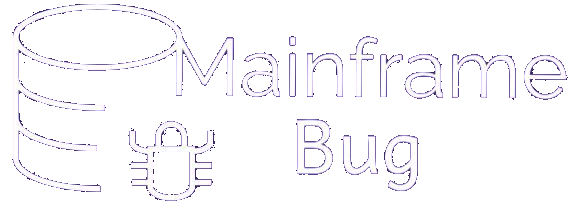## JCL Quiz

### JCL Quiz 1-10

• Q1 - In order to continue a job after a return code of 12 in step1, what the step2 EXEC statement include?
• A - CONTINUE
• B - COND = (12, GT)
• C - COND = (12, GE)
• D - COND = (12 ,LE)
• Answer : B - COND = (12, GT)
• Q2 - What statement marks the beginning of an in-stream or cataloged procedure and assigns default values to parameters defined in the procedure?
• A - STREAM
• B - SET
• C - PROC
• D - PEND
• Answer : C - PROC
• Q3 - What parameter of the job statement is used to limit the CPU time consumed by the job?
• A - RUNTIME
• B - TIME
• C - LIMIT
• D - EXECMAX
• Answer : B - TIME
• Q4 - What is the function of //JCLLIB statement?
• A - Identifies the source of the JCL commands
• B - Points to the system software library
• C - Marks the beginning of the in-stream JCL
• D - Identifies the libraries that the system will search
• Answer : D - Identifies the libraries that the system will search
• Q5 - What is concatenating?
• A - Overriding or adding procedures
• B - Logically connecting input datasets
• C - Overriding a called PROC ddname
• D - All of the above
• Answer : B - Logically connecting input datasets
• Q6 - What are the parameters you can specify in both JOB and EXEC?
• A - REGION
• B - TIME
• C - COND
• D - All of the above
• Answer : D - All of the above
• Q7 - Which statements are not allowed in Procedures?
• A - JOB
• B - JOBLIB
• C - JCLLIB
• D - SYSIN DD
• E - All of the above
• Answer : E - All of the above
• Q8 - How do you choose the block size?
• A - Block size should be multiples of LRECL for fixed files and multiples of LRECL + 4 for variable files.
• B - Block size should be 5 less than LRECL
• C - If LRECL=n then Blocksize = (n+10)/2
• D - If LRECL=n then Blocksize = (n-10)/2
• Answer : A - Block size should be multiples of LRECL for fixed files and multiples of LRECL + 4 for variable files.
• Q9 - How do you specify the restart parameter, if you need to restart from the step STEP1 in the proc EMPPROC ? The proc is invoked in the step EMPPRCS of the job.
• A - RESTART=STEP1.EMPPRCS
• B - RESTART=EMPPRCS.STEP1
• C - RESTART=STEP1 OF EMPPRCS
• D - RESTART.EMPPRCS=STEP1
• Answer : B - RESTART=EMPPRCS.STEP1
• Q10 - What does & and && means in JCL ?
• A - & means temporary parameter ,&& means symbolic dataset.
• B - & means temporary parameter ,&& means sysin dataset.
• C - & means symbolic parameter ,&& means temporary dataset.
• D - & means sysin parameter ,&& means SYSDUMP dataset.
• Answer : C - & means symbolic parameter ,&& means temporary dataset.